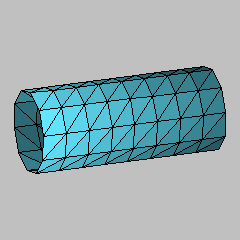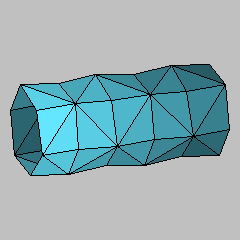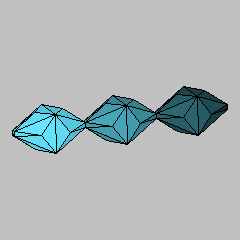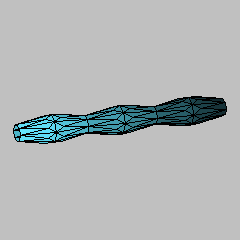## Discrete Cylinders and Delaunay SurfacesElectronic Geometry Model No. 2000.11.041

#### Description

Here we show four discrete constant mean curvature surfaces, the first two of which are discrete analogs of smooth cylinders, and the last two of which are discrete analogs  of smooth Delaunay surfaces . We define discrete constant mean curvature surfaces as piecewise linear continuous triangulated surfaces that are critical for the area functional with respect to all variations through surfaces of the same type that preserve the simplicial structure and the oriented volume to one side of the surface, and we explain this definition in more detail below.

By a discrete surface in Euclidean 3-space, we mean a triangular mesh T, that is, a 2-dimensional simplicial complex, together with a piecewise linear map to Euclidean 3-space. The latter is determined by the images of the vertices.

The area of a discrete surface T is the sum of the Euclidean areas of the triangles in T. One can also define the volume of T when T is orientable: the volume is the oriented volume enclosed by the cone of T over the origin in Euclidean 3-space.

One can consider variations of discrete surfaces: Let Vi be the set of interior vertices of a discrete surface T. A boundary-preserving variation T(t) of T is defined as a C2 variation in t of the vertices in Vi so that the vertices comprise the set Vi when t=0. The straightness of the edges and the flatness of the triangles are preserved as the vertices move.

We say that a compact discrete surface T is of constant mean curvature if it is critical for area with respect to all boundary-preserving variations T(t) with the property that the volume of T(t) is independent of t. In the case that T is noncompact, then we say it is of constant mean curvature when every compact discrete surface contained in it is of constant mean curvature.

This is analogous to the definition of smooth constant mean curvature surfaces. In the case of smooth constant mean curvature surfaces, there exist ones that are periodic surfaces of revolution, called Delaunay surfaces. The cylinder is a special case of them.

Here we show two discrete analogs of smooth cylinders, and an analog of Delaunay surfaces. These surfaces are constructed by positioning the vertices so that the above definition of constant mean curvature is satisfied.

The first discrete constant mean curvature cylinder is obtained by choosing positive reals a and e and an integer k greater than 2, and then choosing the vertices to be

pj,l = (a cos (2 Pi j/k),a sin (2 Pi j/k),e l)

for integers j and l. We then make a grid of rectangular faces, and cut the faces by diagonals with endpoints pj,l and pj+1,l+1. This example has to be a discrete constant mean curvature surface, because its symmetry group is transitive on the vertices. In fact, we could generalize the example by introducing a twist between levels (replacing 2 Pi j/k with 2 Pi j/k + c l) and the same would still hold.

The second example is made by choosing positive reals a, b, e, and an integer k greater than 2, and choosing the vertices to be

pj,l = (a cos(2 Pi j/k),a sin(2 Pi j/k), e l)

when j+l is even, and

pj,l = (b cos(2 Pi j/k),b sin(2 Pi j/k), e l)

when j+l is odd, for integers j and l. We then make a grid of quadrilateral faces, and cut the faces by diagonals with endpoints pj,l and pj+1,l+1 if j+l is even, and by diagonals with endpoints pj,l+1 and pj+1,l if j+l is odd. The vertices with even j+l are of degree eight, and the vertices with odd j+l are of degree four. One can show the existence of unequal values of a and b so that the surface is of constant mean curvature, using an intermediate value argument. For example, one could view a, e, and k as independent parameters, and then b is a dependent parameter which must be fixed so that the surface has constant mean curvature.

The third and fourth examples are discrete Delaunay surfaces in the sense that they are periodic. They can be produced by taking the vertices to be

pj,l = (a cos(2 Pi j/k),a sin(2 Pi j/k), e l)

when l is even, and

pj,l = (b cos(2 Pi j/k),b sin(2 Pi j/k), e l)

when l is odd, for integers j and l. The parameters a, b, e, and k can be chosen freely. We then make a grid of isosceles trapezoidal faces, and put an extra vertex in each of the trapezoidal faces, and connect this extra vertex by edges to each of the four vertices of the surrounding trapezoid. We have found by numerical experimentation  that it is possible to move these extra vertices (out of the trapezoids) to positions so that the following two properties hold:

• the resulting discrete surface has constant mean curvature, and
• the surface has the same symmetry group as the surface that consisted of the grid of isosceles trapezoidal faces.

Model produced with: JavaView version 2.04.001

 Keywords Constant Mean Curvature Surface; Discrete Surface; Periodic Surface; Surface of Revolution MSC-2000 Classification 53-04 (53-XX, 68Uxx, 68Rxx, 65Kxx, 65M50) Zentralblatt No. 01682979

#### References

1. C. Delaunay: Sur la surface de r{\'e}volution, dont la courbure moyenne est constante, J. Math. Pures Appl. 6 (1841), 309--315.
2. Konrad Polthier and Wayne Rossman: Index of Discrete Constant Mean Curvature Surfaces, Preprint 484 TU-Berlin, to appear in: Crelle (2000), http://www-sfb288.math.tu-berlin.de/~konrad/articles.html.
3. Ulrich Pinkall and Konrad Polthier: Computing Discrete Minimal Surfaces and Their Conjugates, Experimental Mathematics 2 (1993), 15--36.

#### Submission information

Submitted: Thu Nov 30 12:50:27 CET 2000.
Revised: Mon Aug 27 11:18:12 CET 2001.
Accepted: Tue Sep 11 15:14:57 CET 2001.

Technische Universität Berlin
Fachbereich Mathematik
Straße des 17. Juni 136
10623 Berlin
Germany
polthier@math.tu-berlin.de